# Using python, opencv obtains and changes pixels, modifies image channels, and trims ROI

2022-01-30 09:43:50

# Use Python,OpenCV obtain 、 Change pixel , Modify the image channel , Tailoring ROI

This blog will introduce the use of Python,OpenCV obtain 、 Change pixel , Modify the image channel , Interested in intercepting images ROI; Single channel diagram ,BGR Three channel diagram , Four channel transparent diagram , Opaque chart ;

## 1. design sketch

Original picture VS Change the lower right pixel to red , Change the upper left corner 1/4 The area is green , The renderings are as follows ：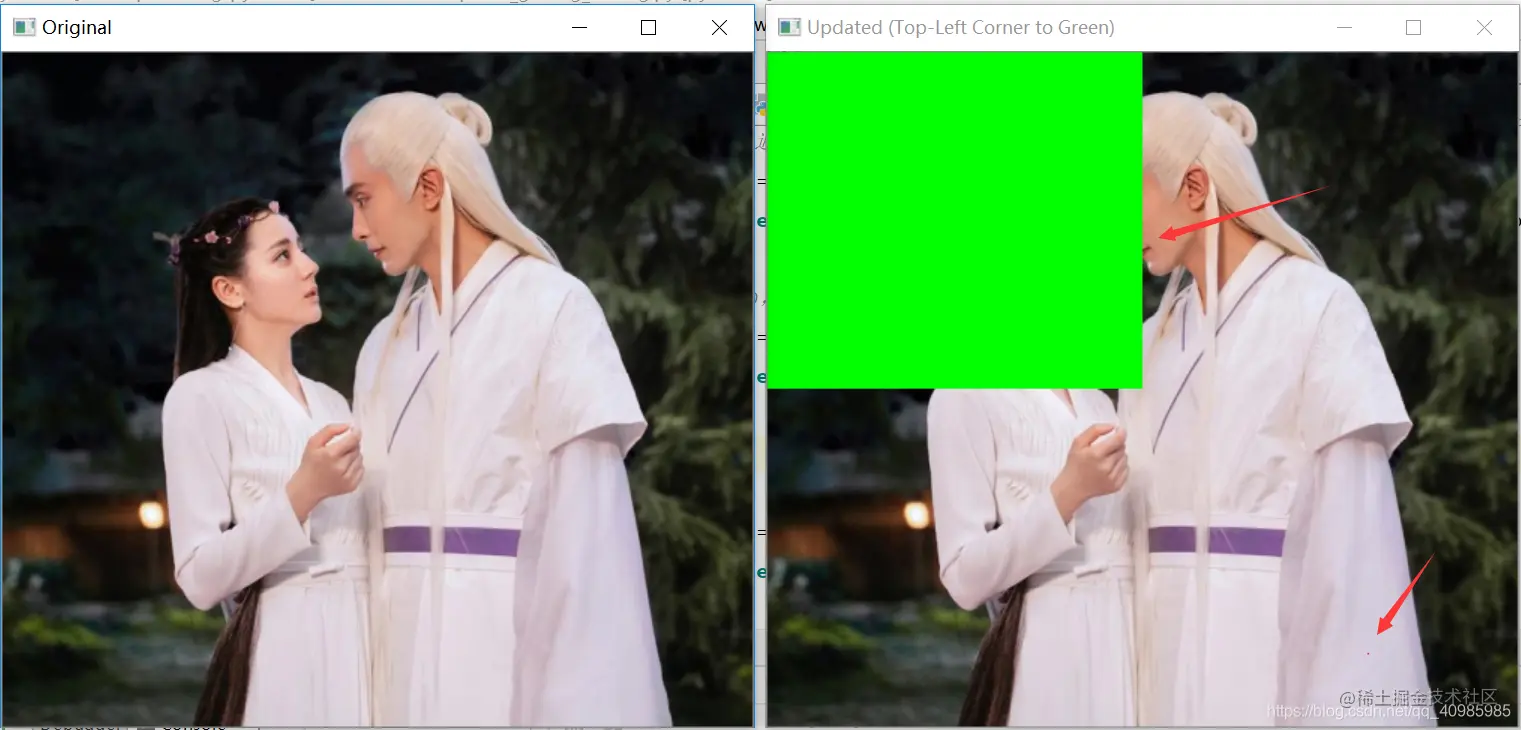Crop the region of interest ： Intercept the upper left corner respectively 、 Upper right corner 、 The lower left corner 、 The lower right corner , Each account 1/4; The renderings are as follows ：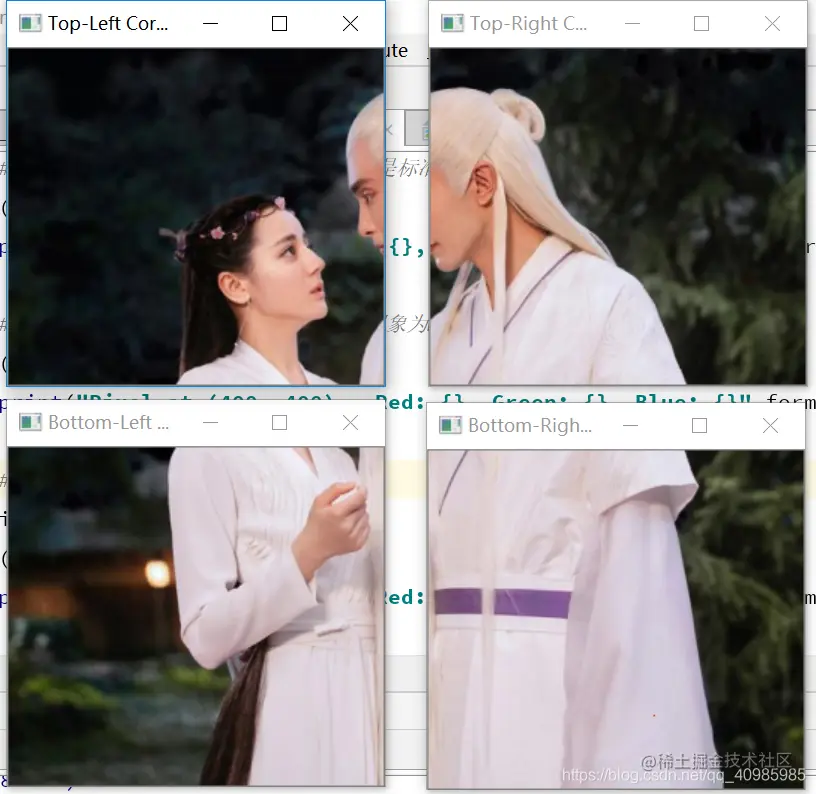Original picture VS The effect of single channel gray image is as follows ：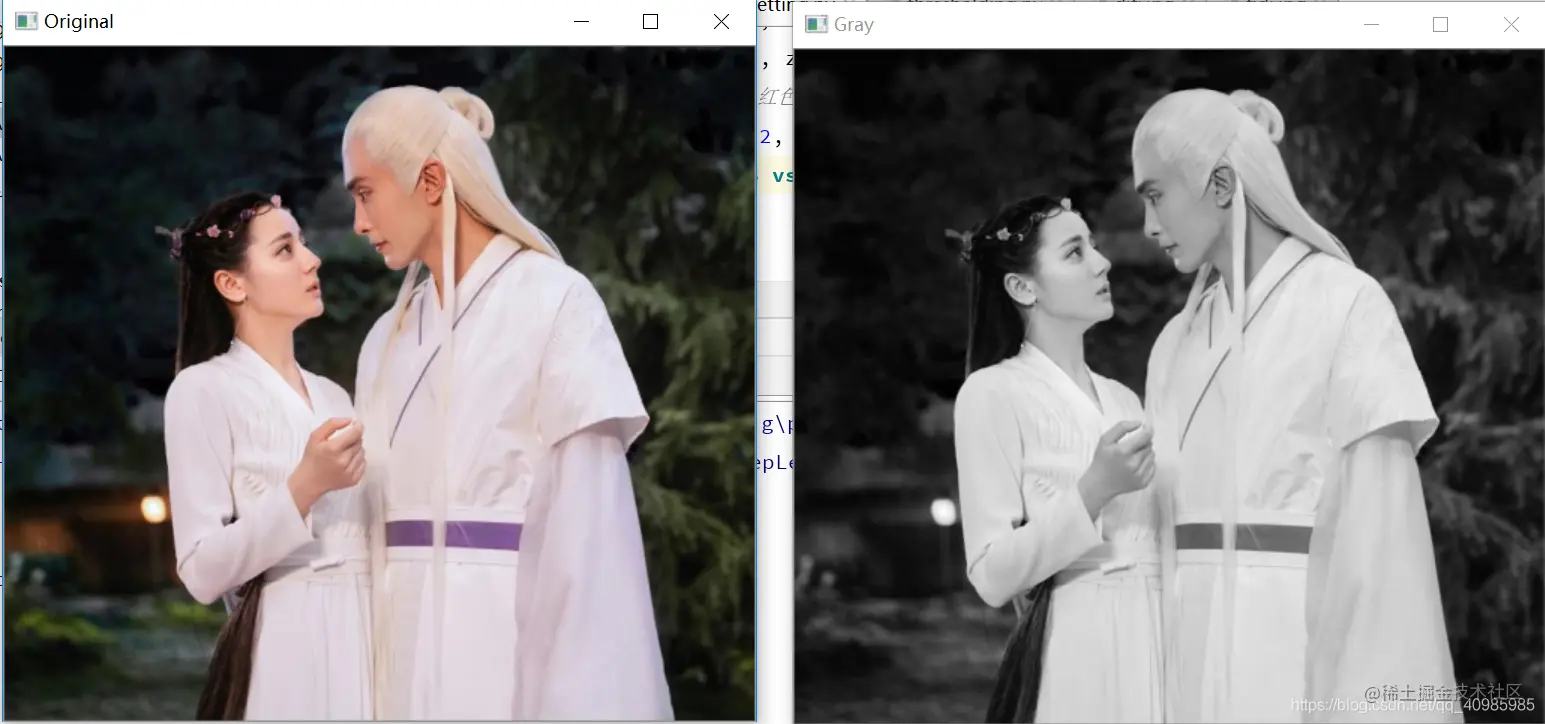Upper left original VS The upper right R Access map VS The lower left G Access map VS The lower right B The effect of the channel diagram is as follows ：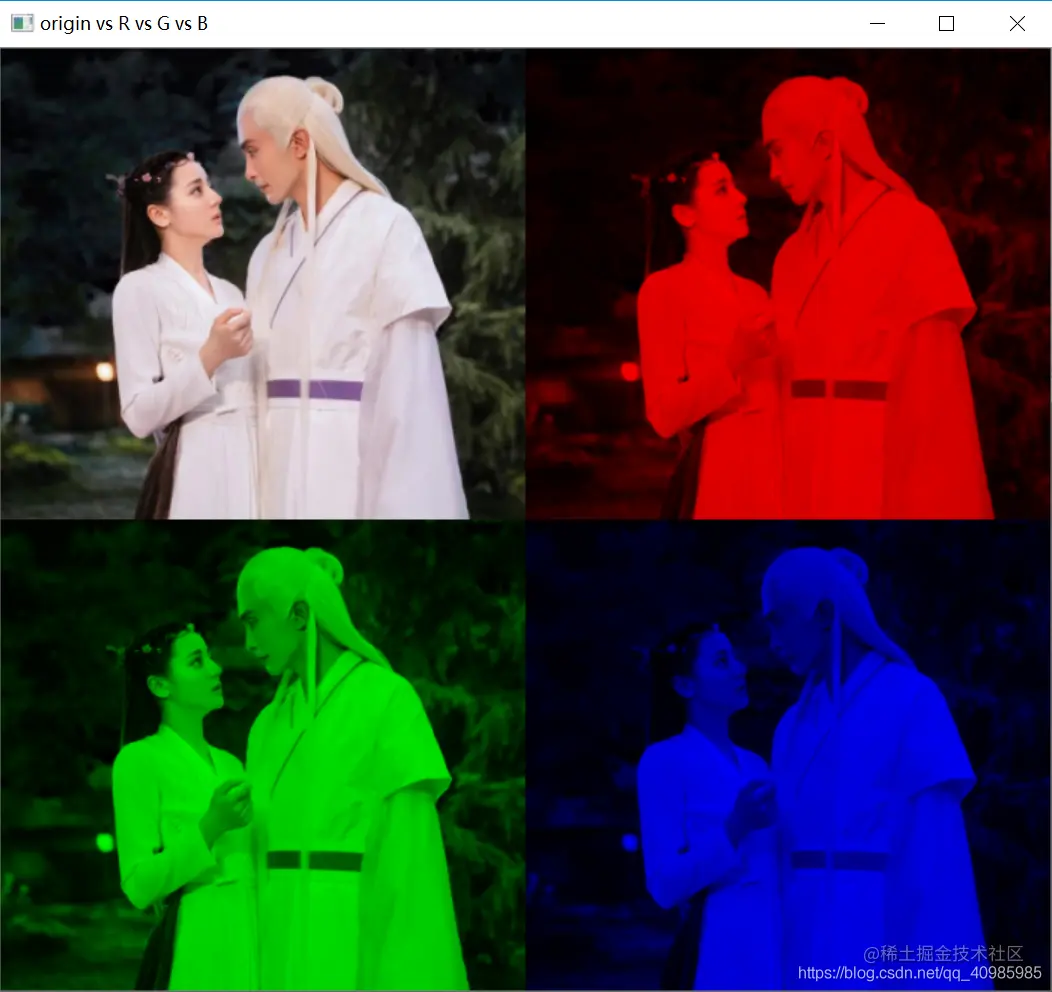Images 4 passageway Full transparency VS Opaque rendering ：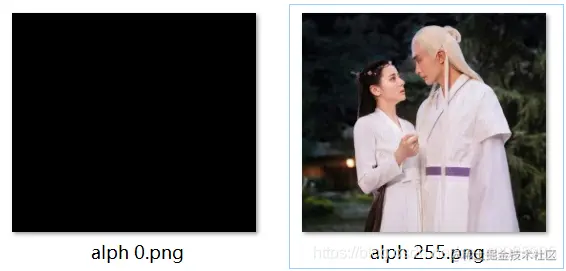## 2. Source code

``````# USAGE
# python opencv_getting_setting.py --image fjdj.png

#  Import necessary packages
import argparse

import cv2
import imutils
import numpy as np

#  Build command line parameters and parse
# --image  Disk picture path , The default name is current py File sibling Directory ：fjdj.jpg

ap = argparse.ArgumentParser()
ap.add_argument("-i", "--image", type=str, default="fjdj.jpg",
help="path to the input image")
args = vars(ap.parse_args())
ap = argparse.ArgumentParser()

#  Load image , Get spatial dimension （ Width 、 Height ）, Show the original image to the screen
image = imutils.resize(image, width=430)
origin = image.copy()
(h, w) = image.shape[:2]
cv2.imshow("Original", image)

#  Image with Numpy Array exists , Get the upper left corner , Image index from 0 Start
#  Image with BGR Channel representation , Because in the beginning BGR It's a standard. , Later it was adjusted to RGB
(b, g, r) = image[0, 0]
print("Pixel at (0, 0) - Red: {}, Green: {}, Blue: {}".format(r, g, b))

#  obtain x=380,y=380 Pixel value , Imagine the image as M*N Matrix ,M For the line ,N Column
(b, g, r) = image[380, 380]
print("Pixel at (380, 380) - Red: {}, Green: {}, Blue: {}".format(r, g, b))

#  to update x=50,y=20 The pixels are red
image[380, 380] = (0, 0, 255)
(b, g, r) = image[380, 380]
print("Pixel at (380, 380) - Red: {}, Green: {}, Blue: {}".format(r, g, b))

#  Calculate the center of the image
(cX, cY) = (w // 2, h // 2)

#  Use the array slice to get the upper left corner 1/4 Part of
tl = image[0:cY, 0:cX]
cv2.imshow("Top-Left Corner", tl)

#  alike , Crop with array slices   Upper right corner 、 The lower left corner 、 Lower right corner , And show
tr = image[0:cY, cX:w]
br = image[cY:h, cX:w]
bl = image[cY:h, 0:cX]
cv2.imshow("Top-Right Corner", tr)
cv2.imshow("Bottom-Right Corner", br)
cv2.imshow("Bottom-Left Corner", bl)

#  Use pixel tiles to change the color of pixel areas
image[0:cY, 0:cX] = (0, 255, 0)

#  Show the picture after updating pixels
cv2.imshow("Updated (Top-Left Corner to Green)", image)

gray = cv2.cvtColor(origin, cv2.COLOR_BGR2GRAY)
cv2.imshow("Gray", gray)

(h, w) = origin.shape[:2]
zeros = np.zeros((h, w), dtype="uint8")
#  take origin Separate into red , Green and blue channels ,  And then we use Numpy  The zero array constructs the representation of each channel separately
(B, G, R) = cv2.split(origin)
R = cv2.merge([zeros, zeros, R])
G = cv2.merge([zeros, G, zeros])
B = cv2.merge([B, zeros, zeros])
cv2.imshow("B G R", np.hstack([B, G, R]))
#  Build the output frame   The original picture is in the upper left corner   The upper right corner of the red channel   The lower right corner of the green channel   The lower left corner of the blue channel
output = np.zeros((h * 2, w * 2, 3), dtype="uint8")
output[0:h, 0:w] = origin
output[0:h, w:w * 2] = R
output[h:h * 2, 0:w] = G
output[h:h * 2, w:w * 2] = B
cv2.imshow("origin vs R vs G vs B", imutils.resize(output, width=700))

alpha0 = np.dstack([origin, np.ones((h, w), dtype="uint8") * 0])
cv2.imshow("alph 0", alpha0)
cv2.imwrite("alph 0.png", alpha0)

alpha1 = np.dstack([origin, np.ones((h, w), dtype="uint8") * 255])
cv2.imshow("alph 255", alpha1)
cv2.imwrite("alph 255.png", alpha1)
cv2.waitKey(0)
Copy code ``````